### Home > A2C > Chapter 4 > Lesson 4.3.1 > Problem4-140

4-140.
1. Sketch the graph of y = 2(x − 1)2 + 4. Homework Help ✎

1. Now rewrite the equation y = 2(x − 1)2 + 4 without parentheses. Remember the order of operations!

2. What would the difference be between the graphs of the two equations above? This is sort of a trick question, but explain your reasoning.

3. What is the parent of y = 2(x − 1)2 + 4?

4. What is the parent of y = 2x2 − 4x + 6?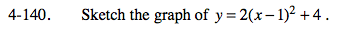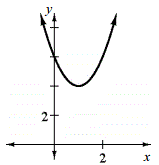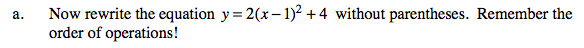y = 2x2 − 4x + 6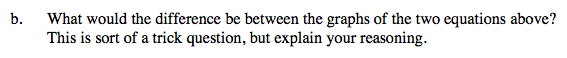Where is the vertex of y = 2(x − 1)2 + 4? Or y = 2x2 − 4x + ?
What are their stretch factors?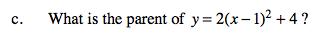Refer to the Math Notes box from Lesson 4.3.2.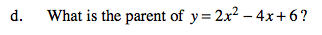See part (c).